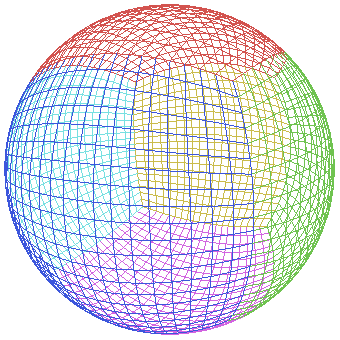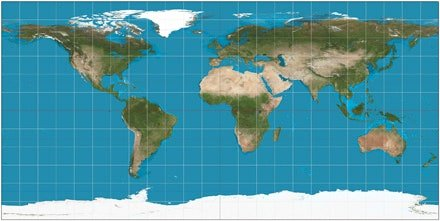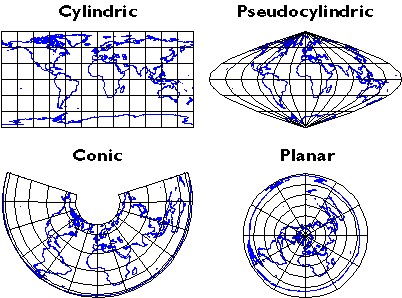Search In
• More options...
Find results that contain...
Find results in...

# Terrain Deformation

In games we think of terrain as a flat plane subdivided into patches, but did you know the Earth is actually round? Scientists say that as you travel across the surface of the planet, a gradual slope can be detected, eventually wrapping all the way around to form a spherical shape! At small scales we can afford to ignore the curvature of the Earth but as we start simulating bigger and bigger terrains this must be accounted for. This is a big challenge. How do you turn a flat square shape into a sphere? One way is to make a "quad sphere", which is a subdivided cube with each vertex set to the same distance from the center:I wanted to be able to load in GIS datasets so we could visualize real Earth data. The problem is these datasets are stored using a variety of projection methods. Mercator projections are able to display the entire planet on a flat surface, but they suffer from severe distortion near the north and south poles. This problem is so bad that most datasets using Mercator projections cut off the data above and below 75 degrees or so:Cubic projections are my preferred method. This matches the quad sphere geometry and allows us to cover an entire planet with minimal distortion. However, few datasets are stored this way:

It's not really feasible to re-map data into one preferred projection method. These datasets are enormous. They are so big that if I started processing images now on one computer, it might take 50 years to finish. We're talking thousands of terabytes of data that can be streamed in, most of which the user will never see even if they spend hours flying around the planet.

There are many other projection methods:How can I make our terrain system handle a variety of projection methods ti display data from multiple sources? This was a difficult problem I struggled with for some time before the answer came to me.

The solution is to use a user-defined callback function that transforms a flat terrain into a variety of shapes. The callback function is used for culling, physics, raycasting, pathfinding, and any other system in which the CPU uses the terrain geometry:

```#ifdef DOUBLE_FLOAT
void Terrain::Transform(void TransformCallback(const dMat4& matrix, dVec3& position, dVec3& normal, dVec3& tangent, const std::array<double, 16>& userparams), std::array<double, 16> userparams)
#else
void Terrain::Transform(void TransformCallback(const Mat4& matrix, Vec3& position, Vec3& normal, Vec3& tangent, const std::array<float, 16>& userparams), std::array<float, 16> userparams)
#endif```

An identical function is used in the terrain vertex shader to warp the visible terrain into a matching shape. This idea is similar to the vegetation system in Leadwerks 4, which simultaneously calculates vegetation geometry in the vertex shader and on the CPU, without actually passing any data back and forth.

`void TransformTerrain(in mat4 matrix, inout vec3 position, inout vec3 normal, inout vec3 tangent, in mat4 userparams)`

The following callback can be used to handle quad sphere projection. The position of the planet is stored in the first three user parameters, and the planet radius is stored in the fourth parameter. It's important to note that the position supplied to the callback is the terrain point's position in world space before the heightmap displacement is applied. The normal is just the default terrain normal in world space. If the terrain is not rotated, then the normal will always be (0,1,0), pointing straight up. After the callback is run the heightmap displacement will be applied to the point, in the direction of the new normal. We also need to calculate a tangent vector for normal mapping. This can be done most easily by taking the original position, adding the original tangent vector, transforming that point, and normalizing the vector between that and our other transformed position.

```#ifdef DOUBLE_FLOAT
void TransformTerrainPoint(const dMat4& matrix, dVec3& position, dVec3& normal, dVec3& tangent, const std::array<double, 16>& userparams)
#else
void TransformTerrainPoint(const Mat4& matrix, Vec3& position, Vec3& normal, Vec3& tangent, const std::array<float, 16>& userparams)
#endif
{
//Get the position and radius of the sphere
#ifdef DOUBLE_FLOAT
dVec3 center = dVec3(userparams, userparams, userparams);
#else
Vec3 center = Vec3(userparams, userparams, userparams);
#endif

//Get the tangent position before any modification
auto tangentposition = position + tangent;

//Calculate the ground normal
normal = (position - center).Normalize();

//Calculate the transformed position
position = center + normal * radius;

//Calculate transformed tangent
auto tangentposnormal = (tangentposition - center).Normalize();
tangentposition = center + tangentposnormal * radius;
tangent = (tangentposition - position).Normalize();
}```

And we have a custom terrain shader with the same calculation defined below:

```#ifdef DOUBLE_FLOAT
void TransformTerrain(in dmat4 matrix, inout dvec3 position, inout dvec3 normal, inout dvec3 tangent, in dmat4 userparams)
#else
void TransformTerrain(in mat4 matrix, inout vec3 position, inout vec3 normal, inout vec3 tangent, in mat4 userparams)
#endif
{
#ifdef DOUBLE_FLOAT
dvec3 tangentpos = position + tangent;
dvec3 tangentnormal;
dvec3 center = userparams.xyz;
#else
vec3 tangentpos = position + tangent;
vec3 tangentnormal;
vec3 center = userparams.xyz;
#endif
//Transform normal
normal = normalize(position - center);

//Transform position
position = center + normal * radius;

//Transform tangent
tangentnormal = normalize(tangentpos - center);
tangentpos = center + tangentnormal * radius;
tangent = normalize(tangentpos - position);
}```

Here is how we apply a transform callback to a terrain:

```#ifdef DOUBLE_FLOAT
std::array<double, 16> params = {};
#else
std::array<float, 16> params = {};
#endif
params = position.x;
params = position.y;
params = position.z;
terrain->Transform(TransformTerrainPoint, params);```

We also need to apply a custom shader family to the terrain material, so our special vertex transform code will be used:

```auto family = LoadShaderFamily("Shaders/CustomTerrain.json");

When we do this, something amazing happens to our terrain:

If we create six terrains and position and rotate them around the center of the planet, we can merge them into a single spherical planet. The edges where the terrains meet don't line up on this planet because we are just using a single heightmap that doesn't wrap. You would want to use a data set split up into six faces:

All our terrain features like texture splatting, LOD, tessellation, and streaming data are retained with this system. Terrain can be warped into any shape to support any projection method or other weird and wonderful ideas you might have.

•7×   Pasted as rich text.   Paste as plain text instead

Only 75 emoji are allowed.

×   Your previous content has been restored.   Clear editor

×   You cannot paste images directly. Upload or insert images from URL.

×
×

• Pages

• #### Support

• Our Picks
• Gallery
• Videos
• Chat
×
• Create New...